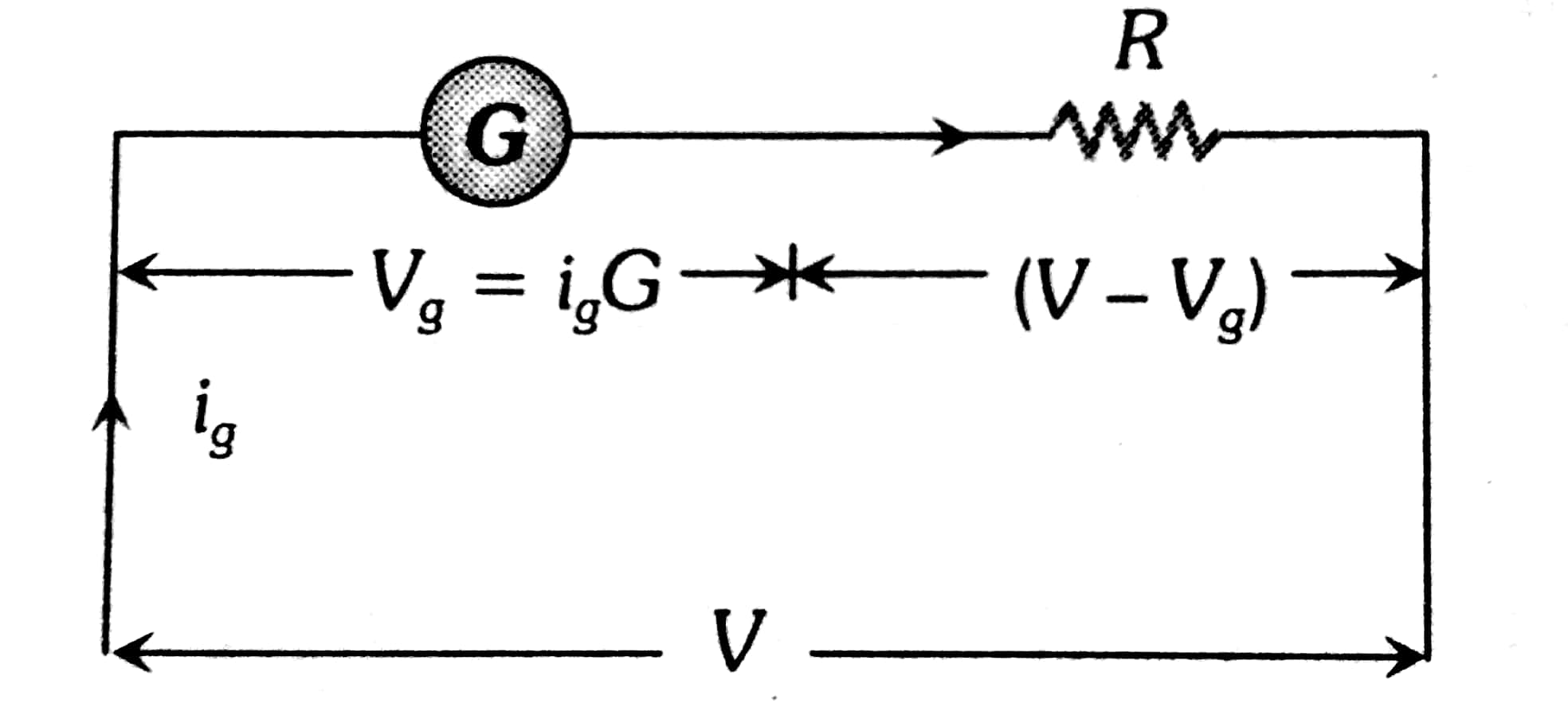# A moving coil galvanometer allows a full  scale current of  A. A series resistance  of 200 is required to convert the above galvanometer into an voltmeter of range0-5 V . Therefore the value of shunt resistance required to convert the above galvanometer into an ammeter of range0-10 mA is : Option 1) Option 2) Option 3) Option 4)

Conversion of galvanometer into voltameter -

Connected a large Resistance   in series

- wherein(R+G) I = V

=>

G=300

Option 1)

Option 2)

Option 3)

Option 4)

Exams
Articles
Questions